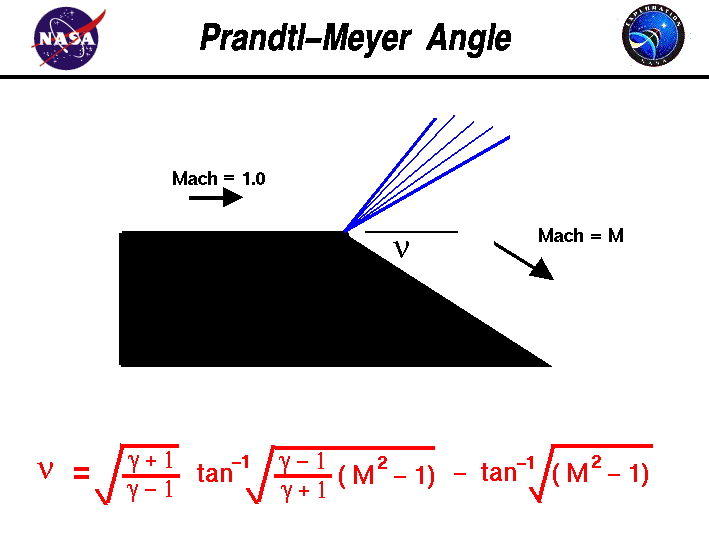+ Text Only Site
+ Non-Flash Version
+ Contact GlennAs a rocket moves through a gas, the gas molecules are deflected around the rocket. If the speed of the rocket is much less than the speed of sound of the gas, the density of the gas remains constant and the flow of gas can be described by conserving momentum and energy. As the speed of the rocket increases towards the speed of sound, we must consider compressibility effects on the gas. The density of the gas varies locally as the gas is compressed by the rocket. When a rocket moves faster than the speed of sound, and there is an abrupt decrease in the flow area, shock waves are generated. If the flow area increases, however, a different flow phenomenon is observed. If the increase is abrupt, we encounter a centered expansion fan.

There are some marked differences between shock waves and expansion fans. Across a shock wave, the Mach number decreases, the static pressure increases, and there is a loss of total pressure because the process is irreversible. Through an expansion fan, the Mach number increases, the static pressure decreases and the total pressure remains constant. Expansion fans are isentropic.

The calculation of the expansion fan involves the use of the Prandtl-Meyer function. This function is derived from conservation of mass, momentum, and energy for very small (differential) deflections. The Prandtl-Meyer function is denoted by the Greek letter nu on the slide and is a function of the Mach number M and the ratio of specific heats gam of the gas.

nu = {sqrt[(gam+1)/(gam-1)]} * atan{sqrt[(gam-1)*(M^2 - 1)/(gam+1)]} - atan{sqrt[M^2 -1]}

where atan is the trigonometric inverse tangent function. It is also written as shown on the slide tan^-1. The meaning of atan can be explained by these two equations:

atan(a) = b

tan(b) = a

As mentioned above, the Mach number of a supersonic flow increases through an expansion fan. The amount of the increase depends on the incoming Mach number and the angle of the expansion. The physical interpretation of the Prandtl-Meyer function is that it is the angle through which you must expand a sonic (M=1) flow to obtain a given Mach number. The value of the Prandtl-Meyer function is therefore called the Prandtl-Meyer angle.

Here's a JavaScript program which solves for the Prandtl-Meyer angle .

# Isentropic Flow Calculator

Input
Output
Mach
Mach Angle
P-M Ang
p/pt
T/Tt
rho/rhot
q/p
A/A*
Wcor/A

You select an input variable by using the choice button labeled Input Variable. Directly below the selection, you then type in the value of the selected variable. When you hit the Enter key on your keyboard, the output values change. The default input variable is the Mach number, and by varying Mach number you can see the effect on Prandtl-Meyer angle. You can also select Prandtl-Meyer angle as an input, and see its effect on the flow variables downstream of the turning.

If you are an experienced user of this calculator, you can use a sleek version of the program which loads faster on your computer and does not include these instructions. You can also download your own copy of the program to run off-line by clicking on this button:

Guided Tours
Activities:

Related Sites:
Rocket Index
Rocket Home
Beginner's Guide Home+ Inspector General Hotline + Equal Employment Opportunity Data Posted Pursuant to the No Fear Act + Budgets, Strategic Plans and Accountability Reports + Freedom of Information Act + The President's Management Agenda + NASA Privacy Statement, Disclaimer, and Accessibility CertificationEditor: Tom Benson NASA Official: Tom Benson Last Updated: May 13 2021 + Contact Glenn